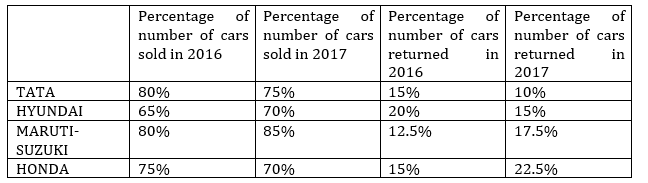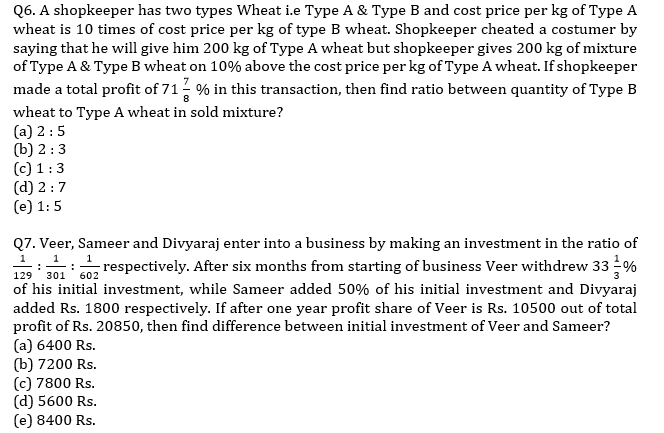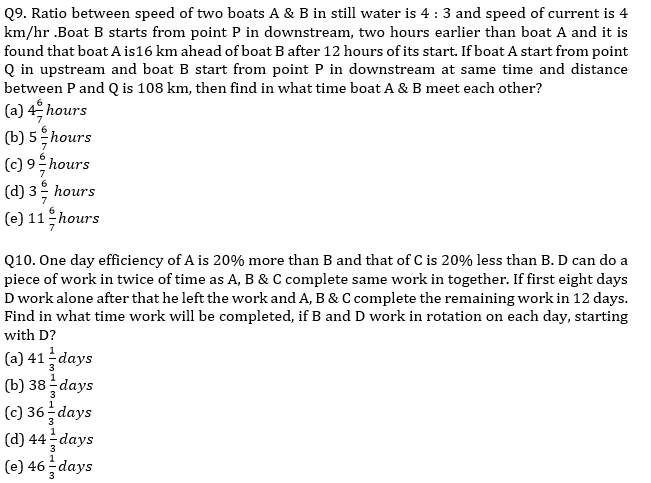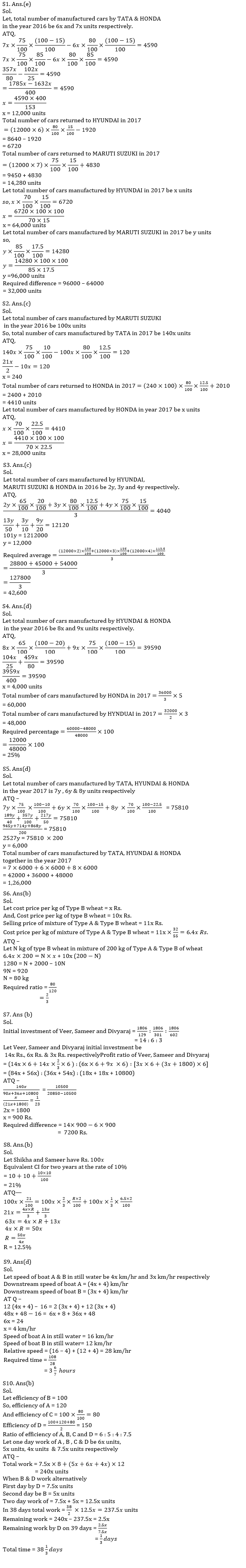Latest Banking jobs   »   Quantitative Aptitude Quiz For RRB PO...   »   Quantitative Aptitude Quiz For RRB PO...

# Quantitative Aptitude Quiz For RRB PO Mains 2021- 11th September

Directions (1 – 5): Given below table shows the percentage of number of cars sold by four different motor companies, percentage of number of total returned cars out of total number of sold cars in two successive years(2016, 2017) . Read the graph carefully and answer the following questions.
Note – (I) Total number of manufactured cars = number of Unsold cars + number of Sold cars
(II) Total actual number of cars sold = Total number of sold cars – number of returned carsQ1. The ratio between total number of cars manufactured by TATA and HONDA in the year 2016 is 6 : 7 and the total actual number of cars sold by HONDA is 4590 units more than that of TATA in the same year. If total number of cars returned to HYUNDAI in the year 2017 is 1920 units less than total number of cars returned to TATA in 2016 and total number of cars returned to MARUTI SUZUKI in the year 2017 is 4830 units more than total number of cars returned to HONDA in 2016, then find the difference between total number of manufactured cars by HYUNDAI and MARUTI SUZUKI in the year 2017?
(a) 36,000
(b) 42,000
(c) 44,000
(d) 40,000
(e) 32,000

Q2. Total number of cars manufactured by TATA in the year 2017 is 40% more than that of total number of cars manufactured by MARUTI SUZUKI in the year 2016 and total number of cars returned to TATA in 2017 is 120 units more than total number of cars returned to MARUTI SUZUKI in the year 2016. If total number of cars returned to HONDA in the year 2017 is 2010 units more than total number of cars returned to MARUTI SUZUKI in the year 2016, then find total number of cars manufactured by HONDA in the year 2017?
(a) 24,000
(b) 20,000
(c) 28,000
(d) 18,000
(e) 16,000

Q3. The ratio between total number of cars manufactured by HYUNDAI, MARUTI SUZUKI & HONDA in the year 2016 is 2 : 3 : 4 and average number of cars returned to these three companies in the same year is 4040 units. If total number of cars manufactured by HYUNDAI, MARUTI SUZUKI & HONDA in the year 2017 is increased by 20%, 25% and 12.5% respectively over that of the previous year, then find the average number of cars manufactured by HYUNDAI, MARUTI SUZUKI & HONDA in the year 2017?
(a) 42,400
(b) 42,800
(c) 42,600
(d) 42,000
(e) 41,600

Q4. The ratio between total number of cars manufactured by HYUNDAI & HONDA in the year 2016 is 8 : 9 and the ratio of number of cars manufactured by HYUNDAI & HONDA in the year 2016 to 2017 is 2 : 3 and 3 : 5 respectively. If total actual number of cars sold by HYUNDAI & HONDA together in the year 2016 is 39590 units then find total number of cars manufactured by HONDA in the year 2017 is what percentage more/less than total number of cars manufactured by HYUNDAI in the same year?
(a) 20%
(b) 15%
(c) 10%
(d) 25%
(e) 35%

Q5. The ratio between total number of cars manufactured by TATA, HYUNDAI & HONDA in the year 2017 is 7 : 6 : 8 and total actual number of cars sold by these companies in the year 2017 is 75810 units, then find total number of cars manufactured by TATA, HYUNDAI & HONDA together in the year 2017?
(a) 1,24,000
(b) 1,25,000
(c) 1,20,000
(d) 1,26,000
(e) 1,36,000Q8. Shikha and Sameer have equal amount. Shikha invested on C.I. for two years at the rate of 10% p.a. and Sameer invested 66 ⅔% of amount at the rate of R% p.a. on simple interest and remaining amount at the rate of 6.5% p.a. on simple interest. If interest received by both at the end of two years are equal, then find the value of ‘R’?
(a) 10%
(b) 12.5%
(c) 15%
(d) 12%
(e) 8%SolutionsClick Here to Register for Bank Exams 2021 Preparation Material#### Congratulations!Download Hindu Review of October 2021: Free PDF